# Texas Go Math Grade 1 Lesson 9.4 Answer Key Equal Amounts

Refer to our Texas Go Math Grade 1 Answer Key Pdf to score good marks in the exams. Test yourself by practicing the problems from Texas Go Math Grade 1 Lesson 9.4 Answer Key Equal Amounts.

## Texas Go Math Grade 1 Lesson 9.4 Answer Key Equal Amounts

Essential Question
Acting out the Problem is a strategy in which students physically act out what is taking place in a word problem. Acting out the Problem can bring the problem to life, helping students to conceptually understand what the task involves and aiding in comprehension and problem-solving.

Unlock the Problem
Blake has 10 pennies. He counts the pennies by twos. What is the total value of the coins?

What information am I given?
Blake has _____ pennies.
Blake counts the pennies by ___.
Blake has 10 pennies.
Blake counts the pennies by 2.

Plan
What is my plan or strategy?
I can ______ the problem.
I can solve the problem.

Solve

Show how you solve the problem.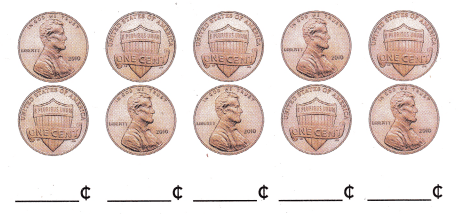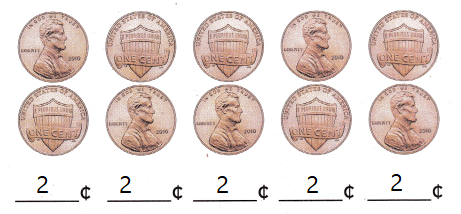Draw a different way to show the value of 10 pennies?Explanation:
1 Nickel = 5 cents
2 x 5 = 10

Home Connection

• Your child used counters to act out the problem. The graphic organizer helps your child analyze the information given in the problem.

Try Another Problem

Act it out to solve. Use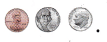Draw and label the coins you use. Write the total.

• What information am I given?
• What is my plan or strategy?

Question 1.
Cameron has 6 nickels. How can he show the same amount in a different way?
1 Nickel = 5¢
6 x 5 = 30¢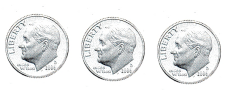Explanation:
the another way is 3 x 10 = 30
1 dime = 10¢

Question 2.
Shelly has 4 dimes. How can she show the same amount ¡n a different way?
1 dime = 10
4 x 10 = 40Explanation:
1 Nickel = 5
5 x 8 = 40

Quarters 3.
David has 5 nickels and I dime. How can he show the same amount ¡n a different way?
5 Nickels
1 Nickel = 5
5 x 5 = 25
1 dime = 10, 25 + 10 = 35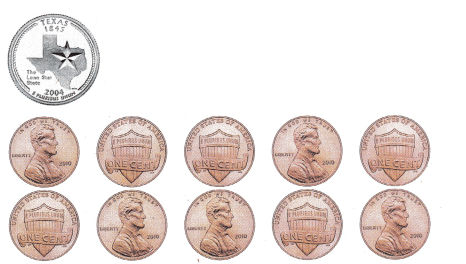Explanation:
The another way is 1 quarter dollar and 10 pennies
25 + 10 = 35

Math Talk
Explain how counting dimes by tens is faster than counting by ones.
Explanation:
If we want to count 100 cents
by dimes we have to count 10 x 10 = 100
By ones we have to count as 1 x 100 = 100

Share and Show

Act it out to solve. UseDraw and label the coins you use. Write the total.

Question 4.
Eva has 5 dimes and 10 pennies. How can she show the same amount in a different way?
5 x 10 = 50
10 x 1 = 10
50 + 10 = 60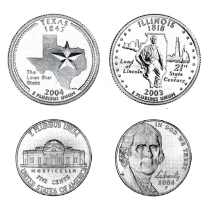Explanation:
Another way is 2 quarter coins and 2 Nickels
2 x 25 = 50
2 x 5 = 10
50 + 10 = 60

Problem Solving

Question 5.
H.O.T. Pam has 5 coins with a total value of 45¢. She gives 2 coins to Choi. What is the total value of the coins Pam has left?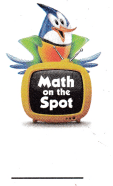Pam has 4 dimes and 1 Nickel
If he gives 2 dimes then the value of the coins is 2 x 10 = 20. 45 – 20 = 25
if he gives 1 dime and 1 nickel; 10 + 5 = 15, 45 – 15 = 30
Explanation:
The answer varies according to the coins.

Question 6.
H.O.T. Multi-Step Mike counted 5 coins by tens. Then he counted 6 coins by twos. Draw and write the total value of the coins.
_____Explanation:
By tens indicates as dimes
By twos indicates as pennies
5 x 10 = 50
3 x 2 = 6
50 + 6 = 56
Total value of coins is 56

Use coins. Choose the correct answer.

Question 7.
Apply Aya saves 30 pennies. How many dimes show the same amount?
(A) 2 dimes
(B) 3 dimes
(C) 1 dime
Explanation:
3 x 10 = 30
1 dime = 10cents

Question 8.
Which coins show the same amount?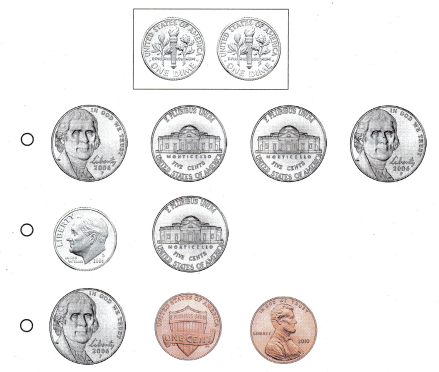Explanation:
1 nickel = 5 cents
5 x 4 = 20
1 dime = 10 cents
2 x 10 = 20

Question 9.
Texas Test Prep Pete has 2 nickels. Which shows the same amount in a different way?Explanation:
1 dime = 10 cents
2 nickels = 5 + 5 = 10

Take Home Activity4 dimes and 5 pennies
Another way4 dimes and 1 nickel

### Texas Go Math Grade 1 Lesson 9.4 Homework and Practice Answer Key

Draw and label coins to solve. Write the total.

Question 1.
Shari has 2 nickels and 3 dimes. How can she show the same amount in a different way?
1 Nickels = 5¢
3 Dimes = 10¢Explanation:
2 x 5 = 10
3 x 10 = 30
10 + 30 = 40¢

Problem Solving

Draw and label coins to solve.

Question 2.
Jason buys an apple for 35¢. He uses 6 coins to pay for the apple. Draw the coins Jason used to pay.Explanation:
1 dime = 10
35 – 10 = 25
1 nickel = 5¢
5 x 5 = 25¢
25 + 10 = 35¢

Texas Test Prep

Lesson Check

Question 3.
Mrs. Washington counts 50 pennies. How many dimes show the same amount?
(A) 10 dimes
(B) 5 dimes
(C) 2 dimes
Explanation:
1 dime = 10 pennies
5 x 10 = 50 pennies.

Question 4.
Which coins show the same amount?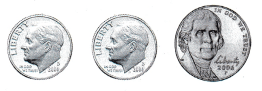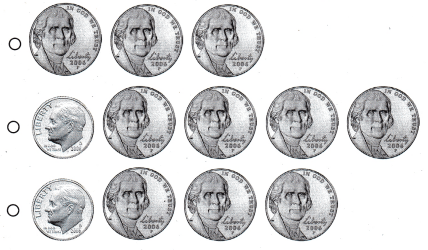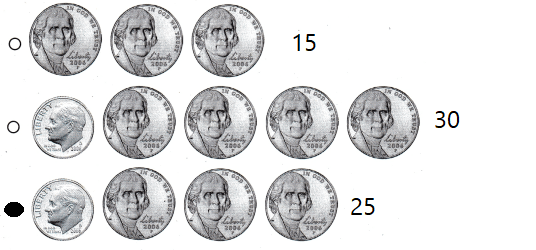Explanation:
25 = 25
1 dime = 10¢
1 Nickel = 5¢

Question 5.
Multi-Step Ted counted 3 coins by tens. He counted 3 coins by fives. Then he counted 6 coins by ones. What ¡s the total value of the coins?
(A) 66¢
(B) 36¢
(C) 51¢